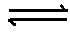Request a call back

<p>a 1.7 % solution of AGNO3 is isotonic with 3.4% solution of glucose.calculate the degree of ddissociation of AGNO3.{88 %}</p>
Asked by PratibhaPandey | 11 May, 2014, 06:47: PMExpert Answer

Let us first calculate observed molar mass of AgNO3.

P(AgNO3) = P(glucose)

and P = w RT

MV

Osmotic pressure of AgNO3

P(AgNO3) = 1.7 RT

MX0.1

P(glucose) = 3.4 RT

MX0.1

1.7 RT = 3.4 RT

MX0.1 180 X0.1

M = 180 X 1.7 = 90

3.4

Normal molar mass of AgNO3 = 169

i = Normal molar mass = 169 = 1.88

Observed molar mass 90

AgNO3 dissociates as:

AgNO3Ag+ + NO3-

If a is the degree of dissociation

Initial moles 1 0 0

Moles after dissociation 1-α α α

Total moles after dissociation = 1-α + α + α = 1 + α

i=1-α + α + α = 1 + α

i=Moles of solute after dissociation = 1+α

Now, 1+α = 1.88

1

α = 1.88 - 1 = 0.88 = 88%

Answered by Vaibhav Chavan | 12 May, 2014, 06:26: PM

## Concept Videos

CBSE 12-science - Chemistry
Asked by KRISHPATEL.soc | 21 Jun, 2021, 05:58: PMANSWERED BY EXPERT
CBSE 12-science - Chemistry
Asked by dhrubajyoti.das | 09 May, 2021, 09:54: PMANSWERED BY EXPERT
CBSE 12-science - Chemistry
Asked by tiwariaatman | 31 Jul, 2020, 05:10: PMANSWERED BY EXPERT
CBSE 12-science - Chemistry
Asked by yogendrasoni142 | 08 Jun, 2020, 05:43: PMANSWERED BY EXPERT
CBSE 12-science - ChemistryAsked by santosh357m | 28 Apr, 2020, 09:28: AMANSWERED BY EXPERT
CBSE 12-science - Chemistry
Asked by Balbir | 27 Jul, 2019, 05:02: PMANSWERED BY EXPERT
CBSE 12-science - Chemistry
Asked by ajaysankhala051 | 04 Jun, 2019, 02:28: PMANSWERED BY EXPERT
CBSE 12-science - Chemistry
Asked by govtsecschoolnayaganv051 | 04 Jun, 2019, 01:30: PMANSWERED BY EXPERT
CBSE 12-science - Chemistry
Asked by Jatinaa786 | 04 Apr, 2019, 05:18: PMANSWERED BY EXPERT
CBSE 12-science - Chemistry
Asked by lekhakarthikeyan | 29 Aug, 2018, 08:08: PMANSWERED BY EXPERT